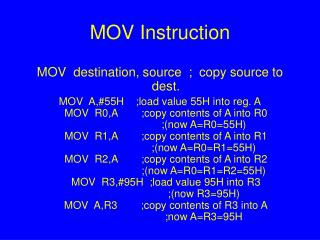DownloadDownload PresentationMOV Instruction

# MOV Instruction

Download Presentation## MOV Instruction

- - - - - - - - - - - - - - - - - - - - - - - - - - - E N D - - - - - - - - - - - - - - - - - - - - - - - - - - -
##### Presentation Transcript

1. MOV Instruction MOV destination, source ; copy source to dest. MOV A,#55H ;load value 55H into reg. AMOV R0,A ;copy contents of A into R0 ;(now A=R0=55H)MOV R1,A ;copy contents of A into R1 ;(now A=R0=R1=55H)MOV R2,A ;copy contents of A into R2 ;(now A=R0=R1=R2=55H)MOV R3,#95H ;load value 95H into R3 ;(now R3=95H)MOV A,R3 ;copy contents of R3 into A ;now A=R3=95H

2. Notes on Programming • Value (proceeded with #) can be loaded directly to registers A, B, or R0 – R7 • MOV R5, #0F9H • If values 0 to F moved into an 8-bit register, the rest assumed all zeros • MOV A, #5 • A too large value causes an error • MOV A, #7F2H

7. Structure of Assembly Language ORG 0H ;start (origin) at location 0 MOV R5,#25H ;load 25H into R5 MOV R7,#34H ;load 34H into R7 MOV A,#0 ;load 0 into A ADD A,R5 ;add contents of R5 to A ;now A = A + R5 ADD A,R7 ;add contents of R7 to A ;now A = A + R7 ADD A,#12H ;add to A value 12H ;now A = A + 12H HERE: SJMP HERE ;stay in this loop END ;end of asm source file

8. Data Types & Directives ORG 500H DATA1: DB 28 ;DECIMAL (1C in Hex) DATA2: DB 00110101B ;BINARY (35 in Hex) DATA3: DB 39H ;HEX ORG 510H DATA4: DB “2591” ; ASCII NUMBERS ORG 518H DATA6: DB “My name is Joe” ;ASCII CHARACTERS

9. Access RAM Locations Using Register Names

10. Access RAM Locations Using Addresses

11. Switch Register Banks

12. Pushing onto Stack

13. Popping from Stack

14. Stack & Bank 1 Conflict

15. Stack & Bank 1 Conflict

16. Arithmetic Instructions and Programs

17. Outlines • Range of numbers in 8051 unsigned data • Addition & subtraction instructions for unsigned data • BCD system of data representation • Packed and unpacked BCD data • Addition & subtraction on BCD data • Range of numbers in 8051 signed data • Signed data arithmetic instructions • Carry & overflow problems & corrections

18. Addition of Unsigned Numbers • ADD A, source ; A = A + source

20. ADDC & Addition of 16-bit Numbers 1 3C E7 3B 8D 78 74 +

21. BCD Number System • Unpacked BCD: 1 byte • Packed BCD: 4 bits

22. Adding BCD Numbers & DA Instruction MOV A,#17H ADD A,#28H MOV A,#47H ;A=47H first BCD operand MOV B,#25H ;B=25 second BCD operand ADD A,B ;hex (binary) addition (A=6CH) DA A ;adjust for BCD addition (A=72H) HEX BCD 29 0010 1001 + 18 + 0001 1000 41 0100 0001 AC=1 + 6 + 0110 47 0100 0111

23. Example

24. Subtraction of Unsigned Numbers • SUBB A, source ; A = A – source – CY • SUBB when CY = 0 • Take 2’s complement of subtraend (source) • Add it to minuend • Invert carry

25. Example (Positive Result)

26. Example (Negative Result)

27. SUBB When CY = 1 • For multibyte numbers

28. Multiplication of Unsigned Numbers • MUL AB ; A  B, place 16-bit result in B and A MOV A,#25H ;load 25H to reg. A MOV B,#65H ;load 65H in reg. B MUL AB ;25H * 65H = E99 where ;B = 0EH and A = 99H

29. Division of Unsigned Numbers • DIV AB ; divide A by B MOV A,#95H ;load 95 into A MOV B,#10H ;load 10 into B DIV AB ;now A = 09 (quotient) and ;B = 05 (remainder)

30. Example ( 1 of 2 )

31. Example ( 2 of 2 )

32. Signed 8-bit Operands • Convert to 2’s complement • Write magnitude of number in 8-bit binary (no sign) • Invert each bit • Add 1 to it

33. Example

34. Example

35. Example

36. Byte-sized Signed Numbers Ranges Decimal Binary Hex -128 1000 0000 80 -127 1000 0001 81 -126 1000 0010 82 …. ………… .. -2 1111 1110 FE -1 1111 1111 FF 0 0000 0000 00 +1 0000 0001 01 +2 0000 0010 02 … ………… ... +127 0111 1111 7F

37. Overflow in Signed Number Operations

38. When Is the OV Flag Set? • Either: there is a carry from D6 to D7 but no carry out of D7 (CY = 0) • Or: there is a carry from D7 out (CY = 1) but no carry from D6 to D7

39. Example

40. Example

41. Example